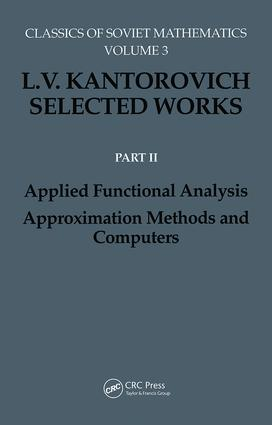# Applied Functional Analysis. Approximation Methods and Computers

## Applied Functional Analysis, Approximation Methods and Computers, 1st Edition

CRC Press

400 pages

##### Purchasing Options:\$ = USD
Hardback: 9782884490139
pub: 1996-02-19
SAVE ~\$85.00
Currently out of stock
\$425.00
\$340.00
x

FREE Standard Shipping!

### Description

This book contains the most remarkable papers of L.V. Kantorovich in applied and numerical mathematics. It explores the principal directions of Kantorovich's research in approximate methods. The book covers descriptive set theory and functional analysis in semi-ordered vector spaces.

### Table of Contents

1. On convergence of the sequence of Bernstein polynomials beyond the principal interval 2. Some remarks on approximation of functions by polynomials with integer coefficients 3. On certain expansions in Bernstein polynomials 4. Explicit representation of a measurable function as the limit of polynomial sequences 5. A direct method for approximate solution of the problem of a double integral minimum 6. On conformal mappings 7. Application of the Stieltjes integral to the calculation of beams lying on elastic supports 8. On approximate calculation of certain types of definite integrals 9. On general methods of improving the convergence for approximate solutions to boundary value problems of mathematical physics 10. On a method of approximate solution of partial differential equations 11. On convergence of variational processes 12. On the convergence of the method of reduction to ordinary differential equations 13. Some remarks on the Ritz method 14. Using the ideas of the Galerkin method in the method of reduction to differential equations 15. On an effective method of solving extremal problems for quadratic functionals 16. On the method of steepest descent 17. On Newton’s method for functional equations 18. Functional analysis and applied mathematics 19. On differential equations of the form xn=f(x) 20. On the Newton Method 21. Some further applications of the Newton method for functional equations 22. New modes of calculations with the tabulator using the binary representations of numbers 23. Prospects in the development and applications of computers 24. Execution of numeric and analytic calculations on computers 25. Increasing of productivity of universal computers in solving problems of mathematical economics

### Subject Categories

##### BISAC Subject Codes/Headings:
MAT037000
MATHEMATICS / Functional Analysis